### IMO Shortlist 1974 problem 11

Kvaliteta:
Avg: 0,0
Težina:
Avg: 0,0
We consider the division of a chess board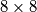$8 \times 8$ in p disjoint rectangles which satisfy the conditions:

a) every rectangle is formed from a number of full squares (not partial) from the 64 and the number of white squares is equal to the number of black squares.

b) the numbers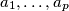$\ a_{1}, \ldots, a_{p}$ of white squares from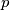$p$ rectangles satisfy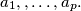$a_1, , \ldots, a_p.$ Find the greatest value of$p$ for which there exists such a division and then for that value of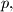$p,$ all the sequences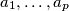$a_{1}, \ldots, a_{p}$ for which we can have such a division.

Moderator says: see http://www.artofproblemsolving.com/Foru ... 41t=58591
Izvor: Međunarodna matematička olimpijada, shortlist 1974